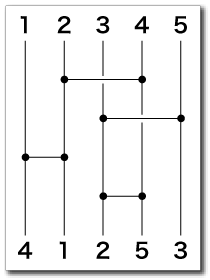Time Limit : sec, Memory Limit : KB

# Drawing Lots

Let's play Amidakuji.

In the following example, there are five vertical lines and four horizontal lines. The horizontal lines can intersect (jump across) the vertical lines.In the starting points (top of the figure), numbers are assigned to vertical lines in ascending order from left to right. At the first step, 2 and 4 are swaped by the first horizontal line which connects second and fourth vertical lines (we call this operation (2, 4)). Likewise, we perform (3, 5), (1, 2) and (3, 4), then obtain "4 1 2 5 3" in the bottom.

Your task is to write a program which reads the number of vertical lines w and configurations of horizontal lines and prints the final state of the Amidakuji. In the starting pints, numbers 1, 2, 3, ..., w are assigne to the vertical lines from left to right.

## Input

w
n
a1,b1
a2,b2
.
.
an,bn


w (w ≤ 30) is the number of vertical lines. n (n ≤ 30) is the number of horizontal lines. A pair of two integers ai and bi delimited by a comma represents the i-th horizontal line.

## Output

The number which should be under the 1st (leftmost) vertical line
The number which should be under the 2nd vertical line
:
The number which should be under the w-th vertical line

## Sample Input

5
4
2,4
3,5
1,2
3,4


## Output for the Sample Input

4
1
2
5
3